####### Welcome to the blog of Positive Mind
About Me | Learn Inredible

### What are operators in Python

In Python and in other programming language operators are special symbols which carry out different Arithmetics or Logical computation.The value that the operator operators on is known as the operand.

For Example:Here, + is an operator for addition. 2 and 3 is an operands and 5 is the output of the operation.

Arithmetic Operators:

Arithmetic Operators are the symbols used to perform mathematical operations such as addition, subtraction, multiplication and division, etc.

Arithmetic Operators in Python

 Operators Meaning Example + Add two operand or unary plus x+y +2 - Subtract two operand from the left or unary minus x-y -2 * Multiply two operands x*y / Divide left operand bt the right one(Always gives answer in float) x/y % Modulus - remainder of the division of left operand by the right x%y (remainder of x/y) // Floor division - division that results into whole number adjusted to the left in the number line x//y ** Exponent - left operand raised to the power of right x**y (x to the power y)

Example#1:Arithmetic Operators in Python: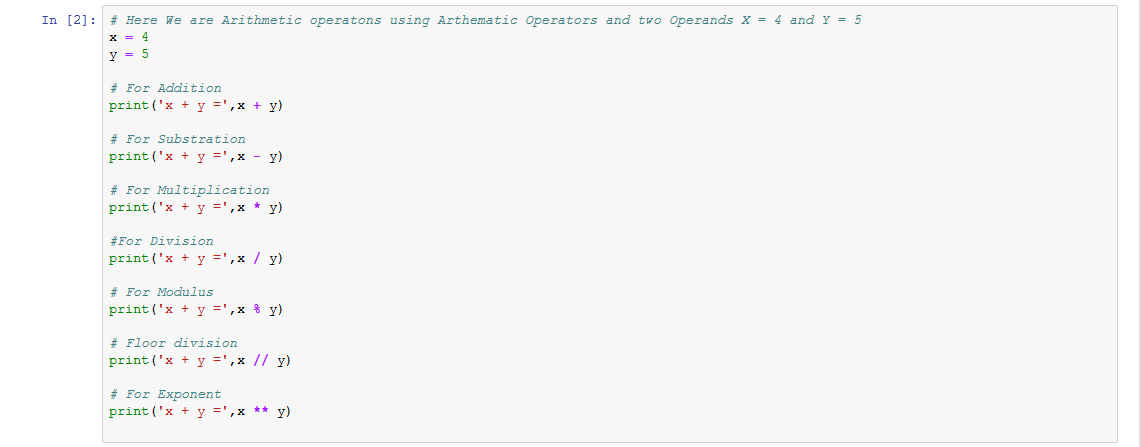When you run the program, the output will be: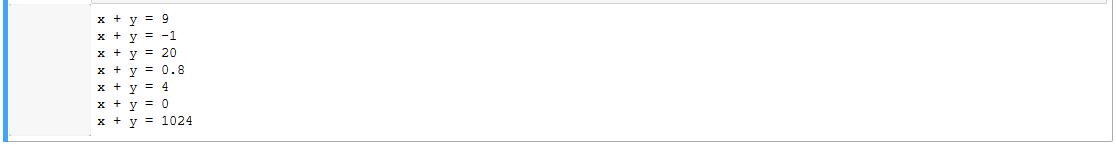## Comparison operators:

Comparison operators in Python are used to compare values. It eithers returns True or False  according to the condition.

Comparison operators in Python

 Operators Meaning Example > Greater that - True if left operand is greater than the right x > y < Less that - True if left operand is less than the right x < y == Equal to - True if both operands are equal x == y != Not equal to - True if operands are not equal x != y >= Greater than or equal to - True if left operand is greater than or equal to the right x >= y <= Less than or equal to - True if left operand is less than or equal to the right x <= y

Example #2: Comparison operators in Python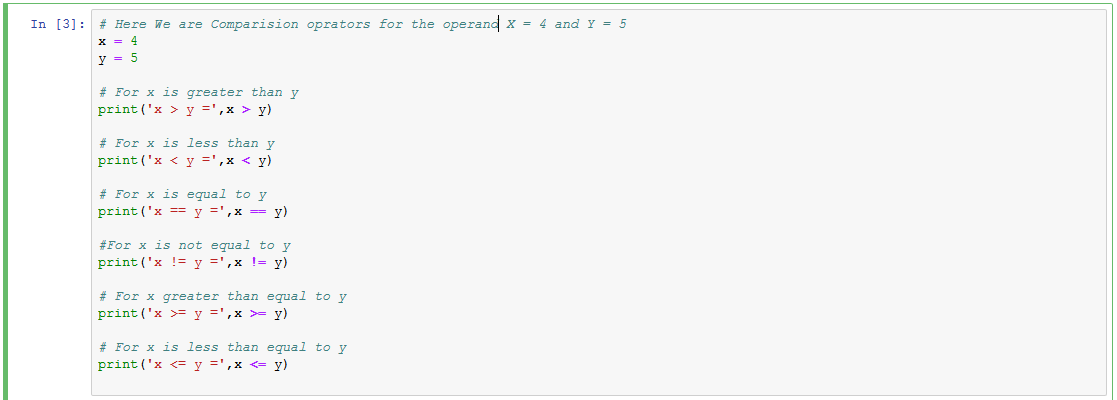When you run the program, the output will be:## Assignment operators:

Assignment operators are used in Python to assign values to variables.

a = 5 is a simple assignment operator that assigns the value 5 on the right to the variable on the left.

There are various compound operators in Python like a += 5 that adds to the variable and later assigns the same. It is equivalent to a = a + 5.

Assignment operators in Python

 Operators Example Equivatent to = x = 5 x = 5 += x += 5 x = x + 5 -= x -= 5 are equal x = x - 5 *= x *= 5 x = x * 5 /= x /= 5 x = x / 5 %= x %= 5 x = x % y //= x //= 5 x = x // 5 **= x **= 5 x = x ** 5 &= x &= 5 x = x & 5 |= x |= 5 x = x | 5 ^= x ^= 5 x = x ^ 5 >>= x >>= 5 x = x >> 5 <<= x <<= 5 X = x << 5

## Special operators:

Python language offers some special type of operators like the identity operator or the membership operator. They are described below with examples.

### Identity operators

is and is not are the identity operators in Python. They are used to check if two values (or variables) are located on the same part of the memory. Two variables that are equal does not imply that they are identical.

Identity operators in Python

 Operator Meaning Example is True if the operands are identical (refer to the same object) x is True Is not True if the operands are not identical (do not refer to the same object) x is not True

### Example #4: Identity operators in Python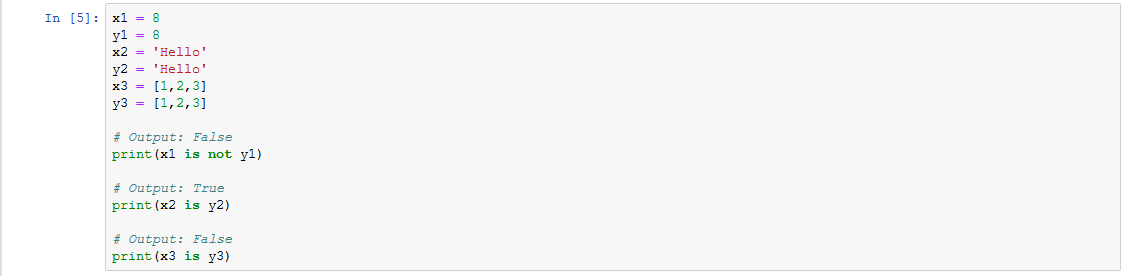When you run the program, the output will be:Here, we see that x1 and y1 are integers of the same values, so they are equal as well as identical. Same is the case with x2 and y2 (strings).

But x3 and y3 are list. They are equal but not identical. It is because interpreter locates them separately in memory although they are equal.

### Membership operators:

in and not in are the membership operators in Python. They are used to test whether a value or variable is found in a sequence (string, list, tuple, set and dictionary).

In a dictionary we can only test for presence of key, not the value.

 Operator Meaning Example in True if value/variable is found in the sequence 5 in x not in True if value/variable is not found in the sequence 5 not in x

### Example #5: Membership operators in Python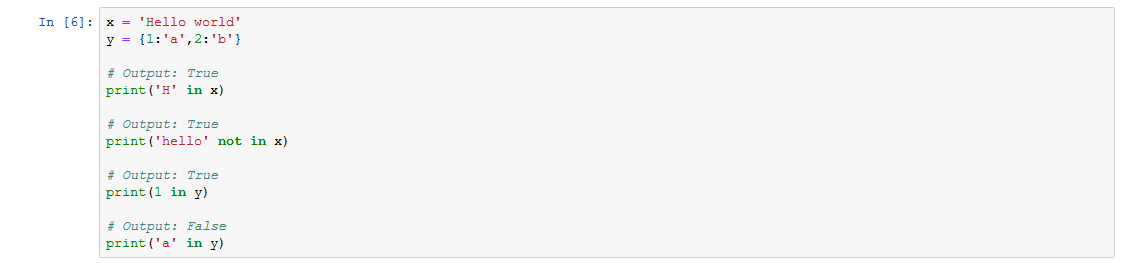When you run the program, the output will be:Here, 'H' is in x but 'hello' is not present in x (remember, Python is case sensitive). Similarly, 1 is key and 'a' is the value in dictionary y. Hence, 'a' in y returns False.

## SUBSCRIBE

Join my mailing list to receive updates on the latest blog posts and other things.